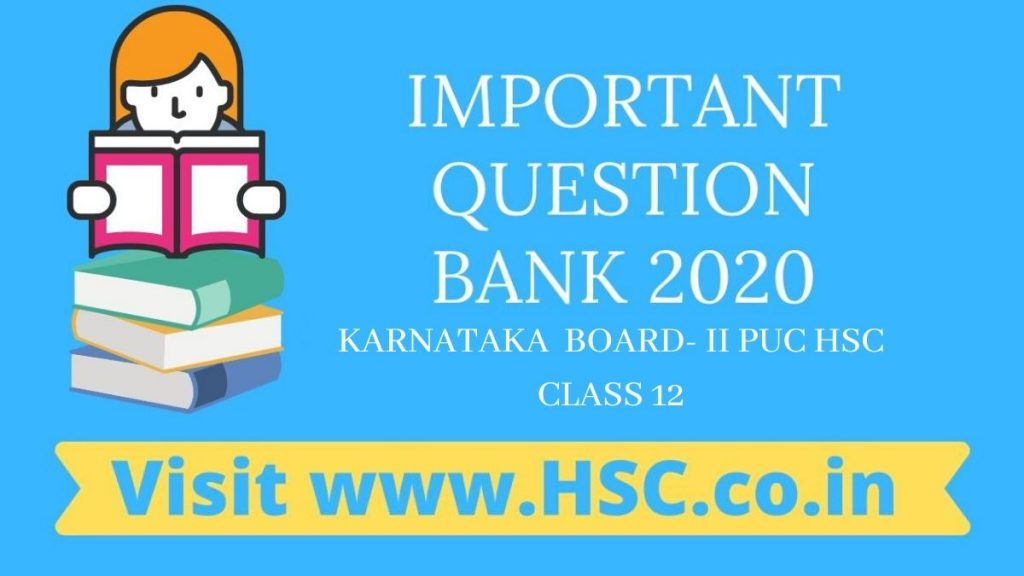# Economics – Important Question Bank for Karnataka Intermediate II PUC (HSC) Board Exam 2020

HSC Board Exams are fast approaching and students are getting anxious about how to prepare for their HSC Board Exams. So we had mentioned some HSC Study Tips to help students in Cracking HSC Exams.

After the tremendous success of our last year Important Question Bank for Karnataka 12th std  PUC HSC Board Exam 2016, 2017, 2018 and 2019 we have also created a list of Important Question Bank for Karnataka Intermediate II PUC (HSC) Board Exam 2020 which are likely to appear in HSC Board Exams this year.To unlock the content Click on any of 3 buttons available in the box below saying “This content is Locked”. Once you click on the button the content will get unlocked on same page itself. You must click on social media button showing in below box ie Facebook, Twitter or Google Plus to unlock the content.

#### ECONOMICS- IMPORTANT QUESTION BANK

1. A consumer wants to consume 2 goods. The price of Bananas is `4 and the price of Mangoes is `’5. The consumer income is `20.  (a) How much bananas can she consume, if entire income is spent on this good?   (b) How much mangoes can she consume, if entire income is spent on this good?   (c) Is the slope of the budget line downward or upward?
2. Briefly explain the functions of RBI.
3. What are the differences between cardinal and ordinal approaches to utility analysis?
4. Explain consumption and investment function with the help of graphs.
5. Explain the short run equilibrium of a monopolist firm, when the cost of production is positive by using TR & TC curves with the help of a diagram.
6. Write a note on balance of payment.
7. Explain the circular flow of income of an economy.
8. Briefly explain in what way Macro Economics is different from Micro Economics
9. Explain the Laws of Returns to scale.
10. ‘Scarcity is the root of all economic problems.’ Explain the statement.
11. Explain the isoquant with the help of the diagram
12. What is monopolistic and perfect competition? Explain the features of perfect competition.
13. Briefly explain the central problems of an economy.
14. Explain open market operations.
15. Explain Keynes’s Consumption Function.
• ##### Visit www.HSC.co.in
16. Explain the role of public expenditure as an instrument of fiscal policy.
17. Suppose demand and supply curves of wheat are as follows: qd = 200 – p and qs = 120 + p.(a) Find the equilibrium price.(b) Find the equilibrium quantity of demand and supply.(c) Find the quantity of demand and supply when p > equilibrium price.(d) Find the quantity of demand and supply when p < equilibrium price.
18. Explain the LDMU with the help of a table and diagram.
19. Explain the derivation of slope of the budget line.
20. Define microeconomic? Explain the various limitations of micro economics.
21. Explain the monetary policy of RBI.
22. Explain the classification of Price Elasticity of Supply with the help of diagrams.
23. What is Income Elasticity of Demand? Calculate the income elasticity of demand when income of a consumer increases from Rs. 10,000 to 12,000 and demand for rice increases from 30 to 40 kgs.
24. Explain the law of diminishing marginal utility.
25. Describe the instruments of fiscal policy.
26. Write any 5 properties of indifference curves.
27. Discuss various methods of measurement of national income.
28. Explain the theories of determination of exchange rate
29. What is money? Explain the functions of money.
30. Discuss the functions of commercial banks
• ##### Visit www.HSC.co.in
31. Discuss various types of short run costs and long run cost.
32. Write a short note on profit maximization of a firm under the following conditions.a) P=MC b) MC must be increasing at q0
33. Write a note on price ceiling and price floor.
34. Explain the expenditure method of measuring national income/GDP.
35. Explain briefly the effects of reforms on Agricultural sector.
36. What are the main causes of unemployment in India?

Hi, we’re trying to collate and gather the data and would be updating it here a few days before the exam. Please keep on visiting our website for updates.

Please use the comments box below and post questions that you think are important from your analysis. It would help the HSC community a lot.

Do subscribe to our updates so that you do not miss out on any important information that we push your way.

Don’t forget to read : MUST REMEMBER THINGS on the day of Exam for HSC Students